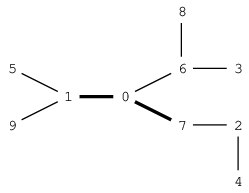Tasks Details
hard
Position speed cameras so as to minimize the lengths of unmonitored paths.
Task Score
100%
Correctness
100%
Performance
100%

Recently, more and more illegal street races have been spotted at night in the city, and they have become a serious threat to public safety. Therefore, the Police Chief has decided to deploy speed cameras on the streets to collect evidence.

There are N+1 intersections in the city, connected by N roads. Every road has the same length of 1. A street race may take place between any two different intersections by using the roads connecting them. Limited by their budget, the police are able to deploy at most K speed cameras on these N roads. These K speed cameras should be installed such that the length of any possible street race route not covered by speed cameras should be as short as possible.

You are given a map of the city in the form of two arrays, A and B of length N, and an integer K:

• For each J (0 ≤ J < N) there is a road connecting intersections A[J] and B[J].

The Police Chief would like to know the minimum length of the longest path out of surveillance after placing at most K speed cameras.

Write a function:

def solution(A, B, K)

that, given arrays A and B of N integers and integer K, returns the minimum length of the longest path unmonitored by speed cameras after placing at most K speed cameras.

For example, given K = 2 and the following arrays:

A = 5 B = 1 A = 1 B = 0 A = 0 B = 7 A = 2 B = 4 A = 7 B = 2 A = 0 B = 6 A = 6 B = 8 A = 6 B = 3 A = 1 B = 9the function should return 2. Two speed cameras can be installed on the roads between intersections 1 and 0 and between intersections 0 and 7. (Another solution would be to install speed cameras between intersections 0 and 7 and between intersections 0 and 6.) By installing speed cameras according the first plan, one of the longest paths without a speed camera starts at intersection 8, passes through intersection 6 and ends at intersection 3, which consists of two roads. (Other longest paths are composed of intersections 5, 1, 9 and 7, 2, 4).

Write an efficient algorithm for the following assumptions:

• N is an integer within the range [1..50,000];
• each element of arrays A, B is an integer within the range [0..N];
• K is an integer within the range [0..N];
• the distance between any two intersections is not greater than 900.
Copyright 2009–2021 by Codility Limited. All Rights Reserved. Unauthorized copying, publication or disclosure prohibited.
Solution
Programming language used Python
Total time used 2 minutes
Effective time used 3 minutes
Notes
not defined yet
Task timeline